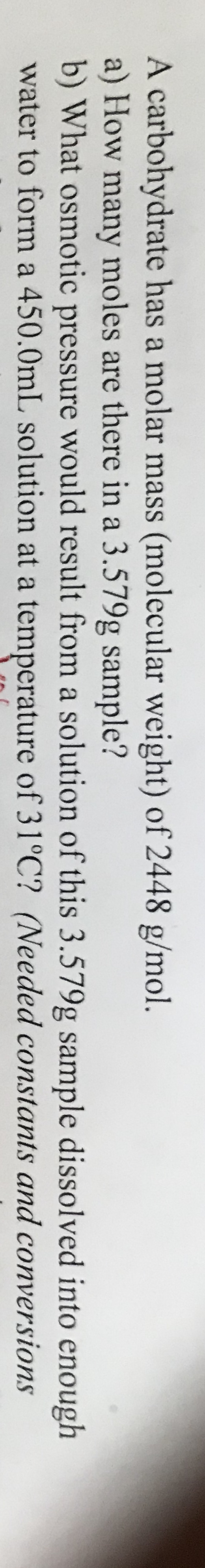# A carbohydrate has a molar mass (molecular weight) of 2448 g/mol.a) How many moles are there in a 3.579g sample?b) What osmotic pressure would result from a solution of this 3.579g sample dissolved into enoughwater to form a 450.0mL solution at a temperature of 31°C? (Needed constants and conversions

Question
132 views

Helphelp_outlineImage TranscriptioncloseA carbohydrate has a molar mass (molecular weight) of 2448 g/mol. a) How many moles are there in a 3.579g sample? b) What osmotic pressure would result from a solution of this 3.579g sample dissolved into enough water to form a 450.0mL solution at a temperature of 31°C? (Needed constants and conversions fullscreen
check_circle

Step 1

Molar mass of given carbohydrate is 2448 g/mol

Step 2

a)

The number of moles of carbohydrate present in 3.579 g sample is,

Step 3

b)

Find the molarity of the given sol...

### Want to see the full answer?

See Solution

#### Want to see this answer and more?

Solutions are written by subject experts who are available 24/7. Questions are typically answered within 1 hour.*

See Solution
*Response times may vary by subject and question.
Tagged in

### Solutions